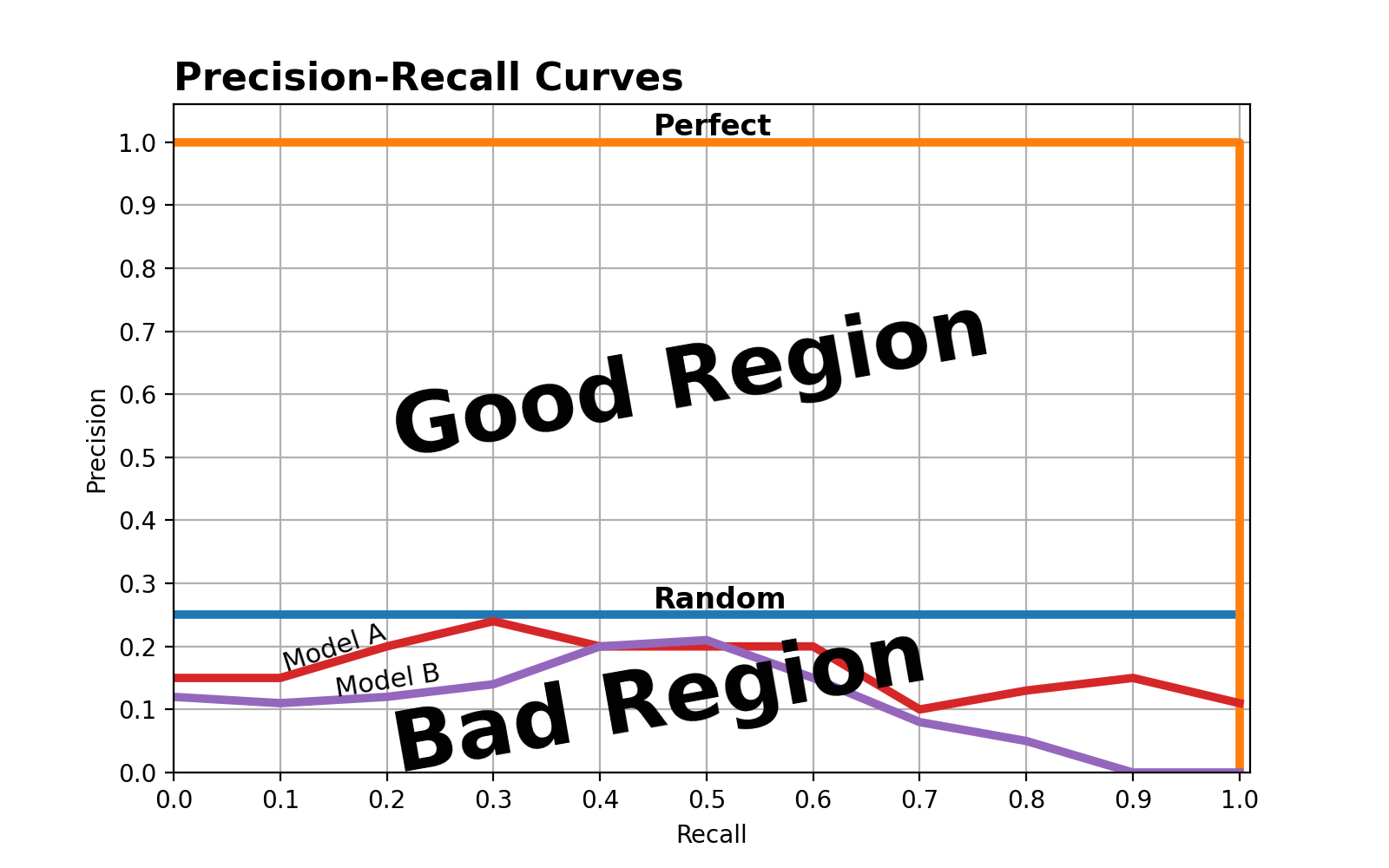# Only Child Solution¶

Neither.

In general, we should select the model with the highest area under the Precision-Recall curve, as higher area under the curve is indicative of better performance. This implies model A is better than model B. But..

Assuming your validation data accurately reflects the U.S. population, about 1 in 4 (25%) of your validation samples should represent an only child. Therefore, a model that randomly guesses whether each person is an only child with 0.25 probability should generate a Precision-Recall curve that mimicks a horizontal line at y = 0.25.This implies that both models A and B are worse than a random model!